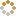﻿ Ningbo University of Technology Online Judge ::  Sum of Sequential Numbers
• ###  Sum of Sequential Numbers

• 时间限制: 1000 ms　内存限制: 65535 K
• 问题描述
• There is no involute formulation concerning factitiously activity of SKB Kontur in this problem. Moreover, there is no formulation at all.
• 输入
• There is the only number N, 1 ≤ N ≤ 10^9.
• 输出
• Your program is to output two positive integers A and P separated with a space such that:
1. N = A + (A + 1) + … + (A + P − 1).
2. You are to choose a pair with the maximal possible value of P.
• 样例输入
• `14`
• 样例输出
• `2 4`
• 提示
• `无`
• 来源
• `HUST`
• 操作
﻿